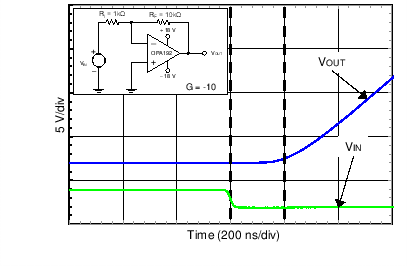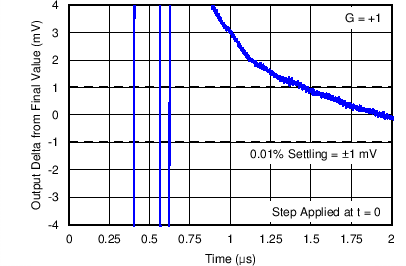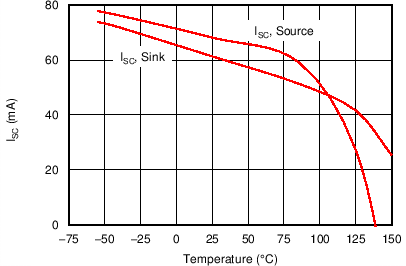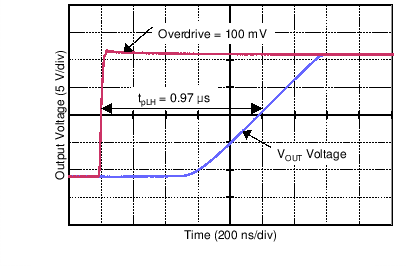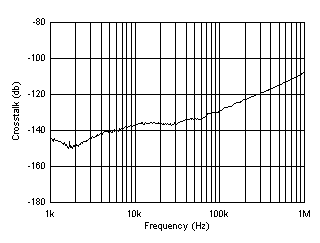SBOS620E December   2013  – November 2015

PRODUCTION DATA.

1. Features
2. Applications
3. Description
4. Revision History
5. Pin Configuration and Functions
6. Specifications
7. Parameter Measurement Information
8. Detailed Description
1. 8.1 Overview
2. 8.2 Functional Block Diagram
3. 8.3 Feature Description
4. 8.4 Device Functional Modes
9. Application and Implementation
1. 9.1 Application Information
2. 9.2 Typical Applications
10. 10Power-Supply Recommendations
11. 11Layout
12. 12Device and Documentation Support
1. 12.1 Device Support
1. 12.1.1 Development Support
2. 12.2 Documentation Support
4. 12.4 Community Resources
6. 12.6 Electrostatic Discharge Caution
7. 12.7 Glossary
13. 13Mechanical, Packaging, and Orderable Information

• D|8
• DGK|8
• DBV|5
• D|8
• DGK|8

6 Specifications

6.1 Absolute Maximum Ratings(1)

over operating free-air temperature range (unless otherwise noted)
MIN MAX UNIT
Supply voltage, VS = (V+) – (V–) ±20
(40, single supply)
V
Signal input pins Voltage Common-mode (V–) – 0.5 (V+) + 0.5 V
Differential (V+) – (V–) + 0.2
Current ±10 mA
Output short circuit(2) Continuous
Temperature Operating range –55 150 °C
Junction 150
Storage, Tstg –65 150
(1) Stresses beyond those listed under Absolute Maximum Ratings may cause permanent damage to the device. These are stress ratings only, which do not imply functional operation of the device at these or any other conditions beyond those indicated under Recommended Operating Conditions. Exposure to absolute-maximum-rated conditions for extended periods may affect device reliability.
(2) Short-circuit to ground, one amplifier per package.

6.2 ESD Ratings

VALUE UNIT
V(ESD) Electrostatic discharge Human-body model (HBM), per ANSI/ESDA/JEDEC JS-001(1) ±4000 V
OPA192
V(ESD) Electrostatic discharge Charged-device model (CDM), per JEDEC specification JESD22-C101(2) ±1000 V
OPA2192
V(ESD) Electrostatic discharge Charged-device model (CDM), per JEDEC specification JESD22-C101(2) ±750 V
OPA4192
V(ESD) Electrostatic discharge Charged-device model (CDM), per JEDEC specification JESD22-C101(2) ±500 V
(1) JEDEC document JEP155 states that 500-V HBM allows safe manufacturing with a standard ESD control process.
(2) JEDEC document JEP157 states that 250-V CDM allows safe manufacturing with a standard ESD control process.

6.3 Recommended Operating Conditions

over operating free-air temperature range (unless otherwise noted)
MIN NOM MAX UNIT
Supply voltage, VS = (V+) – (V–) 4.5 (±2.25) 36 (±18) V
Specified temperature –40 +125 °C

6.4 Thermal Information: OPA192

THERMAL METRIC(1) OPA192 UNIT
D (SOIC) DBV (SOT) DGK (VSSOP)
8 PINS 5 PINS 8 PINS
RθJA Junction-to-ambient thermal resistance 115.8 158.8 180.4 °C/W
RθJC(top) Junction-to-case(top) thermal resistance 60.1 60.7 67.9 °C/W
RθJB Junction-to-board thermal resistance 56.4 44.8 102.1 °C/W
ψJT Junction-to-top characterization parameter 12.8 1.6 10.4 °C/W
ψJB Junction-to-board characterization parameter 55.9 4.2 100.3 °C/W
RθJC(bot) Junction-to-case(bottom) thermal resistance N/A N/A N/A °C/W

6.5 Thermal Information: OPA2192

THERMAL METRIC(1) OPA2192 UNIT
D (SOIC) DGK (VSSOP)
8 PINS 8 PINS
RθJA Junction-to-ambient thermal resistance 107.9 158 °C/W
RθJC(top) Junction-to-case(top) thermal resistance 53.9 48.6 °C/W
RθJB Junction-to-board thermal resistance 48.9 78.7 °C/W
ψJT Junction-to-top characterization parameter 6.6 3.9 °C/W
ψJB Junction-to-board characterization parameter 48.3 77.3 °C/W
RθJC(bot) Junction-to-case(bottom) thermal resistance N/A N/A °C/W

6.6 Thermal Information: OPA4192

THERMAL METRIC(1) OPA4192 UNIT
D (SOIC) PW (TSSOP)
14 PINS 14 PINS
RθJA Junction-to-ambient thermal resistance 86.4 92.6 °C/W
RθJC(top) Junction-to-case(top) thermal resistance 46.3 27.5 °C/W
RθJB Junction-to-board thermal resistance 41.0 33.6 °C/W
ψJT Junction-to-top characterization parameter 11.3 1.9 °C/W
ψJB Junction-to-board characterization parameter 40.7 33.1 °C/W
RθJC(bot) Junction-to-case(bottom) thermal resistance N/A N/A °C/W

6.7 Electrical Characteristics: VS = ±4 V to ±18 V (VS = +8 V to +36 V)

At TA = +25°C, VCM = VOUT = VS / 2, and RLOAD = 10 kΩ connected to VS / 2, unless otherwise noted.
PARAMETER TEST CONDITIONS MIN TYP MAX UNIT
OFFSET VOLTAGE
VOS Input offset voltage ±5 ±25 µV
TA = 0°C to 85°C ±8 ±50
TA = –40°C to +125°C ±10 ±75
VCM = (V+) – 1.5 V ±10 ±40
TA = 0°C to 85°C ±25 ±150
TA = –40°C to +125°C ±50 ±250
dVOS/dT Input offset voltage drift D packages only TA = 0°C to 85°C ±0.1 ±0.5 µV/°C
TA = –40°C to +125°C ±0.15 ±0.8
DBV, DGK, and PW packages only TA = 0°C to 85°C ±0.1 ±0.8
TA = –40°C to +125°C ±0.2 ±1.0
PSRR Power-supply rejection ratio TA = –40°C to +125°C ±0.3 ±1.0 µV/V
INPUT BIAS CURRENT
IB Input bias current ±5 ±20 pA
TA = –40°C to +125°C ±5 nA
IOS Input offset current ±2 ±20 pA
TA = –40°C to +125°C ±2 nA
NOISE
En Input voltage noise (V–) – 0.1 V < VCM < (V+) – 3 V f = 0.1 Hz to 10 Hz 1.30 µVPP
(V+) – 1.5 V < VCM < (V+) + 0.1 V f = 0.1 Hz to 10 Hz 4
en Input voltage noise density (V–) – 0.1 V < VCM < (V+) – 3 V f = 100 Hz 10.5 nV/√Hz
f = 1 kHz 5.5
(V+) – 1.5 V < VCM < (V+) + 0.1 V f = 100 Hz 32
f = 1 kHz 12.5
NOISE (continued)
in Input current noise density f = 1 kHz 1.5 fA/√Hz
INPUT VOLTAGE
VCM Common-mode voltage range (V–) – 0.1 (V+) + 0.1 V
CMRR Common-mode rejection ratio (V–) – 0.1 V < VCM < (V+) – 3 V 120 140 dB
TA = –40°C to +125°C 114 126
(V+) – 1.5 V < VCM < (V+) 100 120
TA = –40°C to +125°C 86 100
(V+) – 3 V < VCM < (V+) – 1.5 V See Typical Characteristics
INPUT IMPEDANCE
ZID Differential 100 || 1.6 MΩ || pF
ZIC Common-mode 1 || 6.4 1013Ω || pF
OPEN-LOOP GAIN
AOL Open-loop voltage gain (V–) + 0.6 V < VO < (V+) – 0.6 V, RLOAD = 2 kΩ 120 134 dB
TA = –40°C to +125°C 114 126
(V–) + 0.3 V < VO < (V+) – 0.3 V, RLOAD = 10 kΩ 126 140
TA = –40°C to +125°C 120 134
FREQUENCY RESPONSE
GBW Unity gain bandwidth 10 MHz
SR Slew rate G = 1, 10-V step 20 V/µs
ts Settling time To 0.01% V S = ±18 V, G = 1, 10-V step 1.4 µs
V S = ±18 V, G = 1, 5-V step 0.9
To 0.001% V S = ±18 V, G = 1, 10-V step 2.1
V S = ±18 V, G = 1, 5-V step 1.8
tOR Overload recovery time VIN × G = VS 200 ns
THD+N Total harmonic distortion + noise G = 1, f = 1 kHz, VO = 3.5 VRMS 0.00008%
Crosstalk OPA2192 and OPA4192, at dc 150 dB
OPA2192 and OPA4192, f = 100 kHz 130
OUTPUT
VO Voltage output swing from rail Positive rail No load 5 15 mV
RLOAD = 10 kΩ 95 110
RLOAD = 2 kΩ 430 500
Negative rail No load 5 15
RLOAD = 10 kΩ 95 110
RLOAD = 2 kΩ 430 500
ISC Short-circuit current ±65 mA
ZO Open-loop output impedance f = 1 MHz, IO = 0 A, see Figure 31 375 Ω
POWER SUPPLY
IQ Quiescent current per amplifier IO = 0 A 1 1.2 mA
TA = –40°C to +125°C, IO = 0 A 1.5
TEMPERATURE
Thermal protection(1) 140 °C

6.8 Electrical Characteristics: VS = ±2.25 V to ±4 V (VS = +4.5 V to +8 V)

At TA = +25°C, VCM = VOUT = VS / 2, and RLOAD = 10 kΩ connected to VS / 2, unless otherwise noted.
PARAMETER TEST CONDITIONS MIN TYP MAX UNIT
OFFSET VOLTAGE
VOS Input offset voltage VCM = (V+) – 3 V ±5 ±25 µV
TA = 0°C to 85°C ±8 ±50
TA = –40°C to +125°C ±10 ±75
(V+) – 3.5 V < VCM < (V+) – 1.5 V See Common-Mode Voltage Range section
VCM = (V+) – 1.5 V ±10 ±40 µV
TA = 0°C to 85°C ±25 ±150
TA = –40°C to +125°C ±50 ±250
dVOS/dT Input offset voltage drift VCM = (V+) – 3 V,
D packages only
TA = 0°C to 85°C ±0.1 ±0.5 µV/°C
TA = –40°C to +125°C ±0.15 ±0.8
VCM = (V+) – 3 V,
DBV, DGK, and PW packages only
TA = 0°C to 85°C ±0.1 ±0.8
TA = –40°C to +125°C ±0.2 ±1.1
VCM = (V+) – 1.5 V, TA = –40°C to +125°C ±0.5 ±3
PSRR Power-supply rejection ratio TA = –40°C to +125°C, VCM = VS / 2 – 0.75 V ±1 µV/V
INPUT BIAS CURRENT
IB Input bias current ±5 ±20 pA
TA = –40°C to +125°C ±5 nA
IOS Input offset current ±2 ±20 pA
TA = –40°C to +125°C ±2 nA
NOISE
En Input voltage noise (V–) – 0.1 V < VCM < (V+) – 3 V, f = 0.1 Hz to 10 Hz 1.30 µVPP
(V+) – 1.5 V < VCM < (V+) + 0.1 V, f = 0.1 Hz to 10 Hz 4
en Input voltage noise density (V–) – 0.1 V < VCM < (V+) – 3 V f = 100 Hz 10.5 nV/√Hz
f = 1 kHz 5.5
(V+) – 1.5 V < VCM < (V+) + 0.1 V f = 100 Hz 32
f = 1 kHz 12.5
in Input current noise density f = 1 kHz 1.5 fA/√Hz
INPUT VOLTAGE
VCM Common-mode voltage range (V–) – 0.1 (V+) + 0.1 V
CMRR Common-mode rejection ratio (V–) – 0.1 V < VCM < (V+) – 3 V 94 110 dB
TA = –40°C to +125°C 90 104
(V+) – 1.5 V < VCM < (V+) 100 120
TA = –40°C to +125°C 84 100
(V+) – 3 V < VCM < (V+) – 1.5 V See Typical Characteristics
INPUT IMPEDANCE
ZID Differential 100 || 1.6 MΩ || pF
ZIC Common-mode 1 || 6.4 1013Ω || pF
OPEN-LOOP GAIN
AOL Open-loop voltage gain (V–) + 0.6 V < VO < (V+) – 0.6 V, RLOAD = 2 kΩ 110 120 dB
TA = –40°C to +125°C 100 114
(V–) + 0.3 V < VO < (V+) – 0.3 V, RLOAD = 10 kΩ 110 126
TA = –40°C to +125°C 110 120
FREQUENCY RESPONSE
GBW Unity gain bandwidth 10 MHz
SR Slew rate G = 1, 10-V step 20 V/µs
ts Settling time To 0.01% VS = ±3 V, G = 1, 5-V step 1 µs
tOR Overload recovery time VIN× G = VS 200 ns
Crosstalk OPA2192 and OPA4192, at dc 150 dB
OPA2192 and OPA4192, f = 100 kHz 130
OUTPUT
VO Voltage output swing from rail Positive rail No load 5 15 mV
RLOAD = 10 kΩ 95 110
RLOAD = 2 kΩ 430 500
Negative rail No load 5 15
RLOAD = 10 kΩ 95 110
RLOAD = 2 kΩ 430 500
ISC Short-circuit current ±65 mA
ZO Open-loop output impedance f = 1 MHz, IO = 0 A, see Figure 31 375 Ω
POWER SUPPLY
IQ Quiescent current per amplifier IO = 0 A 1 1.2 mA
TA = –40°C to +125°C 1.5
TEMPERATURE
Thermal protection(1) 140 °C
(1) For a detailed description of thermal protection, see the Thermal Protection section.

Table 1. Table of Graphs

DESCRIPTION FIGURE
Offset Voltage Production Distribution Figure 1 to Figure 6
Offset Voltage Drift Distribution Figure 7 to Figure 10
Offset Voltage vs Temperature Figure 11
Offset Voltage vs Common-Mode Voltage Figure 12 to Figure 14
Offset Voltage vs Power Supply Figure 15
Open-Loop Gain and Phase vs Frequency Figure 16
Closed-Loop Gain and Phase vs Frequency Figure 17
Input Bias Current vs Common-Mode Voltage Figure 18
Input Bias Current vs Temperature Figure 19
Output Voltage Swing vs Output Current (maximum supply) Figure 20
CMRR and PSRR vs Frequency Figure 21
CMRR vs Temperature Figure 22
PSRR vs Temperature Figure 23
0.1-Hz to 10-Hz Noise Figure 24
Input Voltage Noise Spectral Density vs Frequency Figure 25
THD+N Ratio vs Frequency Figure 26
THD+N vs Output Amplitude Figure 27
Quiescent Current vs Supply Voltage Figure 28
Quiescent Current vs Temperature Figure 29
Open Loop Gain vs Temperature Figure 30
Open Loop Output Impedance vs Frequency Figure 31
Small Signal Overshoot vs Capacitive Load (100-mV Output Step) Figure 32, Figure 33
No Phase Reversal Figure 34
Small-Signal Step Response (100 mV) Figure 37, Figure 38
Large-Signal Step Response Figure 39
Settling Time Figure 40 to Figure 43
Short-Circuit Current vs Temperature Figure 44
Maximum Output Voltage vs Frequency Figure 45
Propagation Delay Rising Edge Figure 46
Propagation Delay Falling Edge Figure 47
Crosstalk vs Frequency Figure 48

6.10 Typical Characteristics

At TA = 25°C, VS = ±18 V, VCM = VS / 2, RLOAD = 10 kΩ connected to VS / 2, and CL = 100 pF, unless otherwise noted.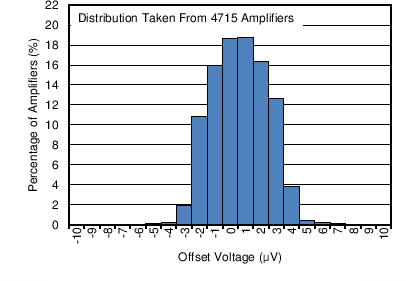Figure 1. Offset Voltage Production Distribution at 25°C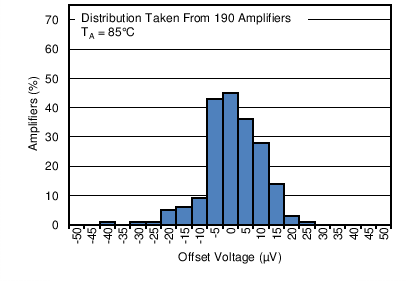Figure 3. Offset Voltage Production Distribution at 85°C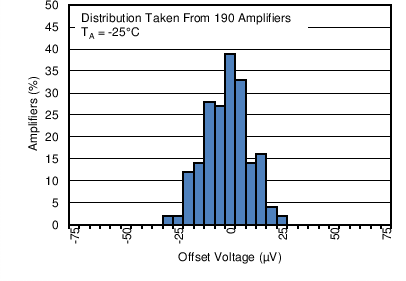Figure 5. Offset Voltage Production Distribution at –25°C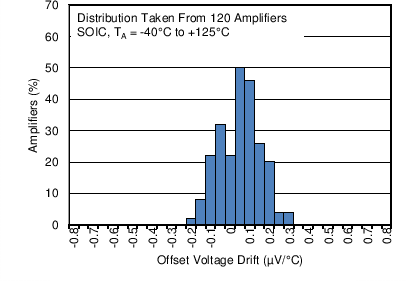OPA192ID and OPA2192ID
Figure 7. Offset Voltage Drift Distribution
from –40°C to +125°C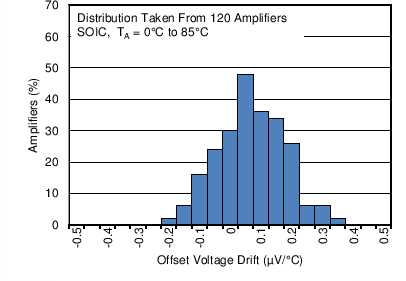OPA192ID and OPA2192ID
Figure 9. Offset Voltage Drift Distribution
from 0°C to 85°C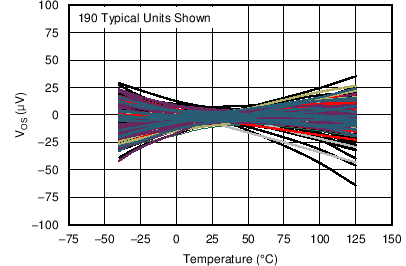Figure 11. Offset Voltage vs TemperatureFigure 13. Offset Voltage vs Common-Mode Voltage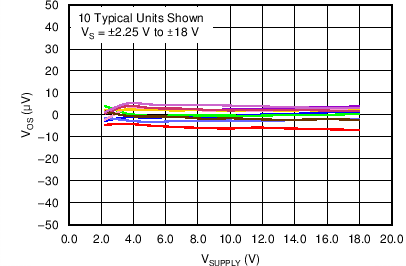Figure 15. Offset Voltage vs Power Supply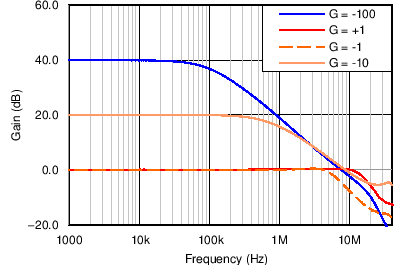Figure 17. Closed-Loop Gain and Phase vs Frequency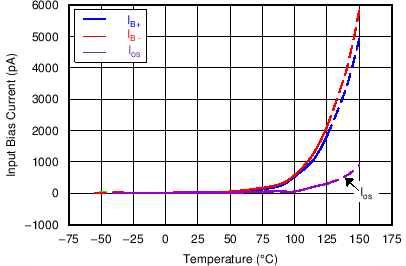Figure 19. Input Bias Current vs Temperature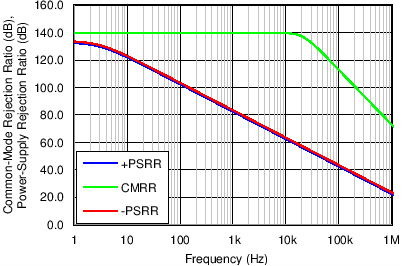Figure 21. CMRR and PSRR vs FrequencyFigure 23. PSRR vs Temperature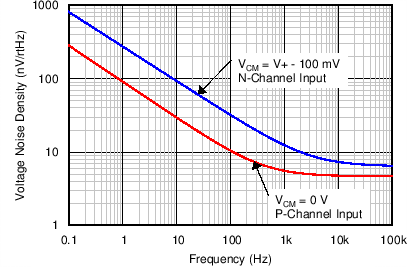Figure 25. Input Voltage Noise Spectral Density
vs Frequency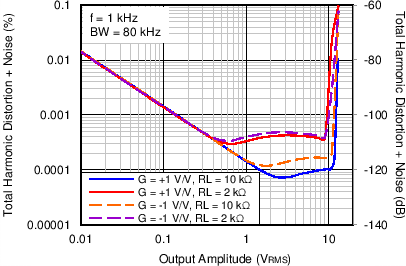Figure 27. THD+N vs Output Amplitude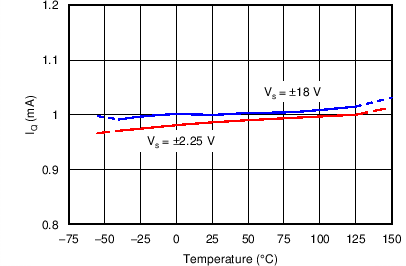Figure 29. Quiescent Current vs TemperatureFigure 31. Open-Loop Output Impedance vs FrequencyFigure 33. Small-Signal Overshoot vs Capacitive Load
(100-mV Output Step)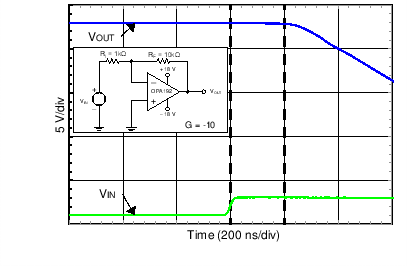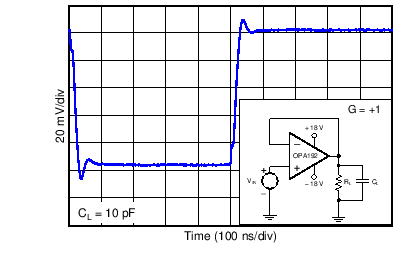Figure 37. Small-Signal Step Response (100 mV)Figure 39. Large-Signal Step Response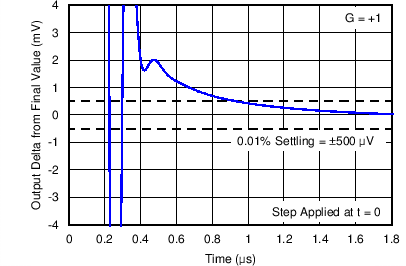Figure 41. Settling Time (5-V Positive Step)Figure 43. Settling Time (5-V Negative Step)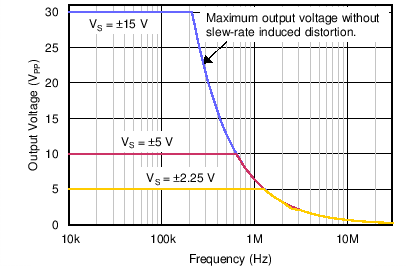Figure 45. Maximum Output Voltage vs Frequency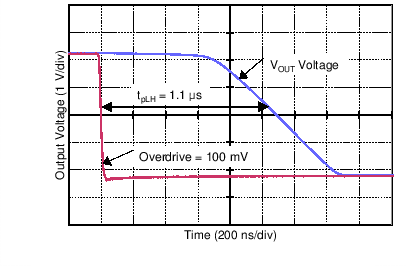Figure 47. Propagation Delay Falling Edge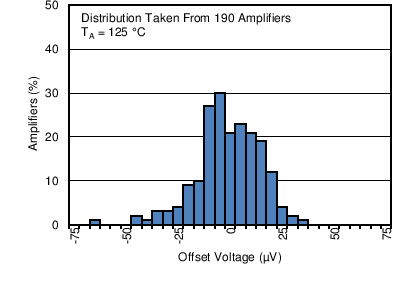Figure 2. Offset Voltage Production Distribution at 125°C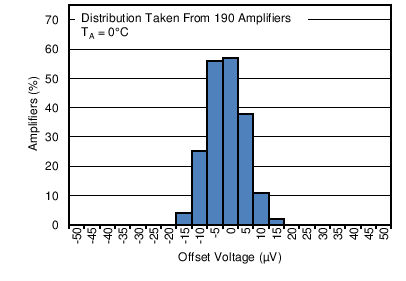Figure 4. Offset Voltage Production Distribution at 0°C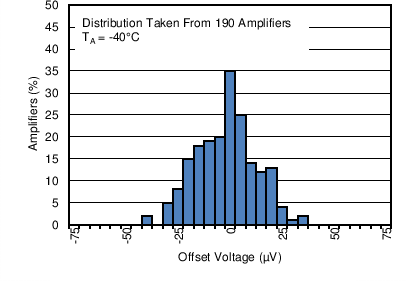Figure 6. Offset Voltage Production Distribution at –40°C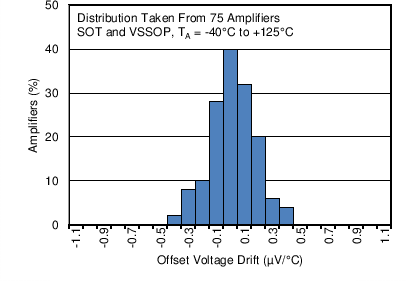OPA192IDBV, OPA192IDGK, OPA2192IDGK, and OPA4192IPW
Figure 8. Offset Voltage Drift Distribution
from –40°C to +125°C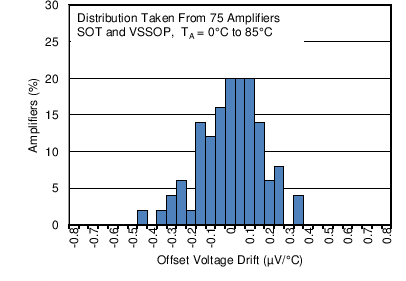OPA192IDBV, OPA192IDGK, OPA2192IDGK, and OPA4192IPW
Figure 10. Offset Voltage Drift Distribution
from 0°C to 85°C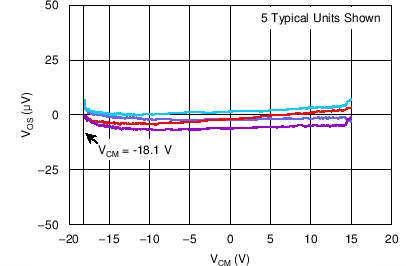Figure 12. Offset Voltage vs Common-Mode Voltage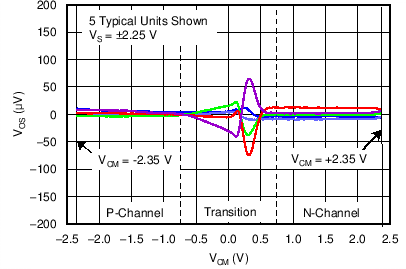Figure 14. Offset Voltage vs Common-Mode Voltage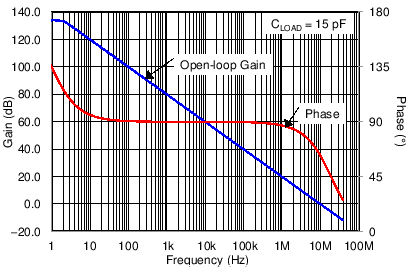Figure 16. Open-Loop Gain and Phase vs Frequency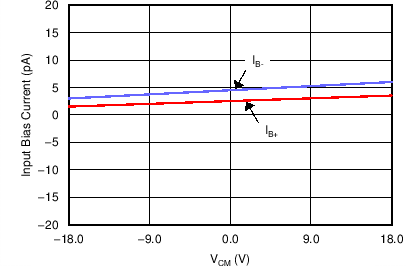Figure 18. Input Bias Current vs Common-Mode Voltage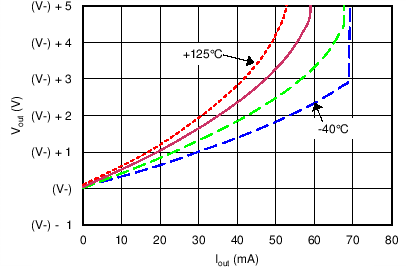Figure 20. Output Voltage Swing vs Output Current (Maximum Supply)Figure 22. CMRR vs Temperature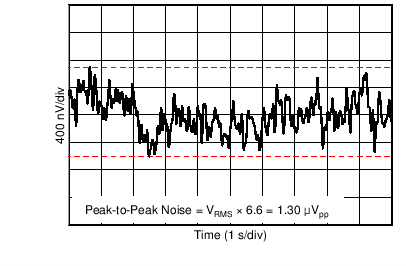Figure 24. 0.1-Hz to 10-Hz Noise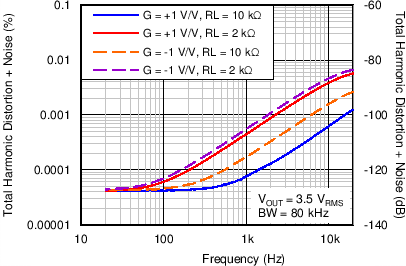Figure 26. THD+N Ratio vs Frequency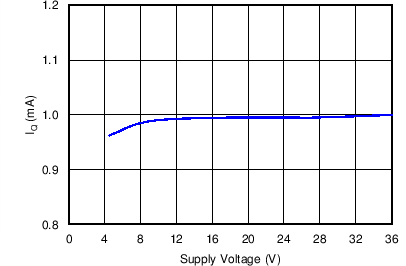Figure 28. Quiescent Current vs Supply Voltage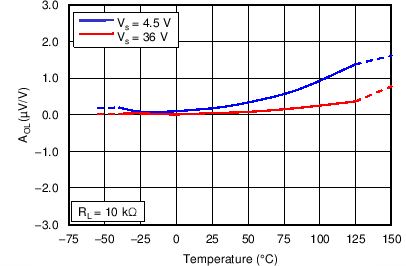Figure 30. Open-Loop Gain vs Temperature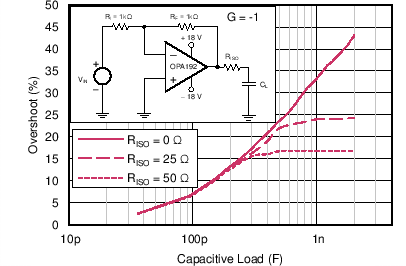Figure 32. Small-Signal Overshoot vs Capacitive Load
(100-mV Output Step)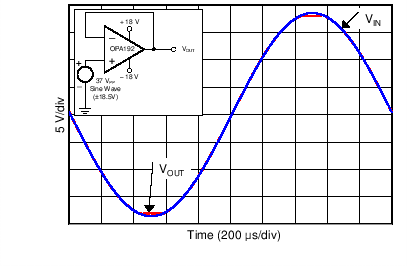Figure 34. No Phase Reversal